# Pluriharmonic function

Jump to: navigation, search

A function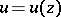ofcomplex variables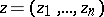in a domainof the complex space,, that has continuous derivatives with respect to the coordinates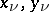,,, inup to the second order inclusive and that satisfies the following system of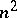equations in: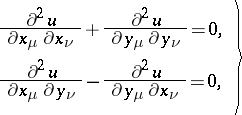(1)One uses the formal derivatives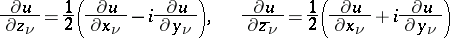to write (1) in the more compact form:(2)

The significance of the class of pluriharmonic functions is determined by the fact that the real and imaginary parts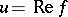and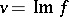of any holomorphic function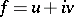inare pluriharmonic functions in; two such real-valued pluriharmonic functions are called conjugate. Conversely, if one is given a pluriharmonic functionin a simply-connected neighbourhoodof a point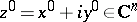, then there exists a holomorphic functioninwith real part equal to. The task of determining this holomorphic functionamounts to finding the conjugate pluriharmonic functionfrom the formula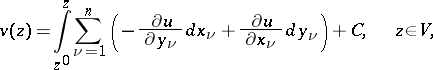where the integral is independent of the path by virtue of (1).

In general, one may also consider complex-valued pluriharmonic functions, which are defined as solutions to (1) or (2). For, the pluriharmonic functions constitute a proper subclass of the class of multiharmonic functions (cf. Multiharmonic function), which in turn is a proper subclass of the class of harmonic functions (cf. Harmonic function); all three classes coincide for. On the other hand, the real-valued pluriharmonic functions constitute a proper subclass of the class of plurisubharmonic functions (cf. Plurisubharmonic function) for, which in turn foris a proper subclass of the class of subharmonic functions (cf. Subharmonic function).

Pluriharmonic functions forhave not only the general properties of harmonic functions, but also characteristic ones due, in general, to the fact that (1), or (2), is an overdetermined system in that case. Suppose, for example, that the pluriharmonic functionin the unit polydisc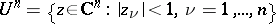is continuous in the closed polydisc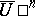,. Then even its boundary values on the skeleton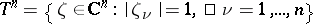, this being a proper part of the entire boundary, cannot be specified as an arbitrary continuous function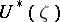,; they must satisfy certain additional conditions. Therefore, within the class of pluriharmonic functions the Dirichlet problem with data on the skeleton is solvable only for special choices of the boundary data .